# IB DP Physics: HL复习笔记7.3.6 Feynman Diagrams & Confinement

### Feynman Diagrams

• Feynman diagrams are a clear way of representing particle interactions in terms of their incoming and outgoing particles and exchange particles
• Although there are many variations of Feynman diagrams, they follow a set of rules:
• The vertical axis represents space
• The horizontal axis represents time
• Gauge bosons are represented by a wavy or dashed lines or a helix
• All other particles are represented by straight lines
• Each line (apart from neutral gauge bosons) must have an arrow with its direction forward in time
• The total charge, baryon number, and lepton number must be conserved at each vertex
• Only two particles may go into/out of a vertex
• Particle lines must not cross over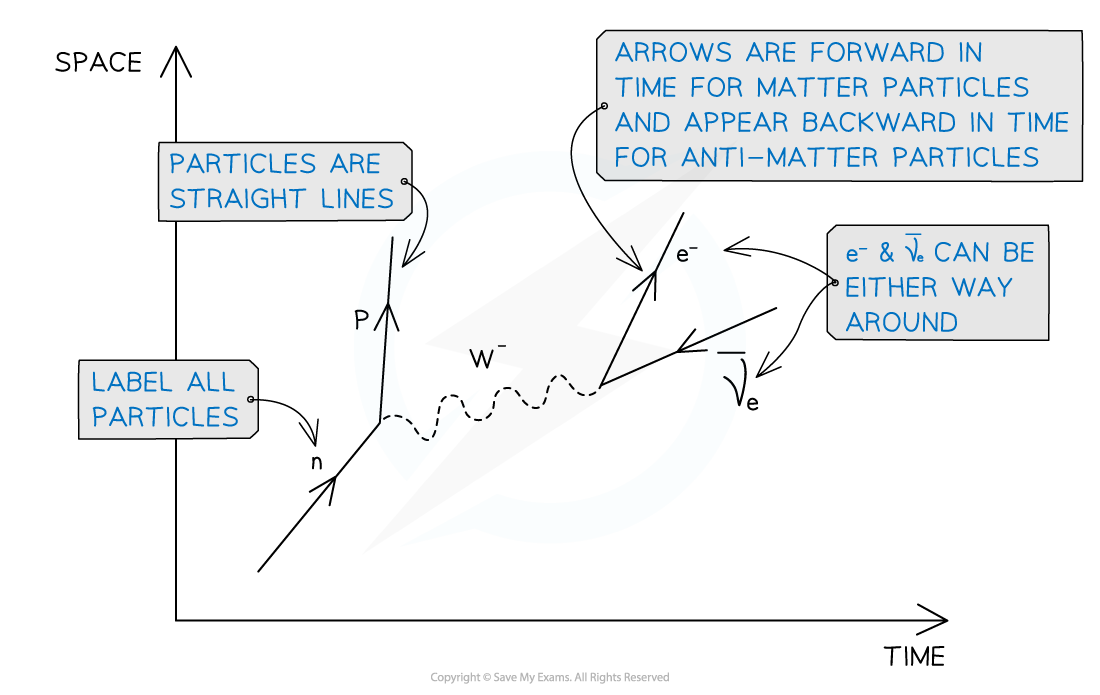Feynman diagrams follow a set of rules which are needed to interpret them accurately

• Feynman diagrams show nothing about the actual path of the particles, so the angles in the diagram are of no significance

#### Exchange Particles

• Gauge bosons are represented differently in each Feynman diagram depending on the type of interaction
• Charged exchange particles (W+ and Wmust have their direction indicated
• Representing exchange particles:
• In the weak interaction, W and Z bosons are represented by a dashed line
• The W+ and W must have an arrow showing their direction (left or right)
• Photons are represented by a wavy line
• Gluons are represented by a helixThe gauge bosons are depicted by a wavy or dashed line, or a helix depending on the type of interaction

#### Worked Example

A neutron interacts with a neutrino in the following way: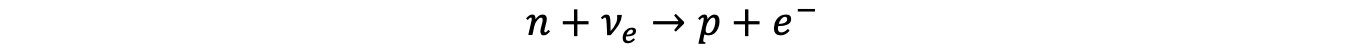Draw the Feynman diagram that corresponds to the neutron and neutrino interaction represented in the equation.• The vertical axis represents space and the horizontal axis represents time
• All particles are labeled correctly
• All particles are represented by straight lines and arrows pointing forward in time
• W+ boson represented by a dashed line with an arrow pointing towards the neutron-proton vertex

#### Exam Tip

The most common exam mistakes when asked to draw Feynman diagrams are missing out arrows indicating the direction of charged gauge bosons or particles. Although you are not required to sketch and label the space and time axes, all particles must be labelled accurately.

#### Quark Transformation in β decay

• β decay occurs because of the weak interaction between quarks

#### Quark Composition: β– decay

• β decay is when a neutron turns into a proton emitting an electron and anti-electron neutrino
• More specifically, a neutron turns into a proton because a down quark turns into an up quark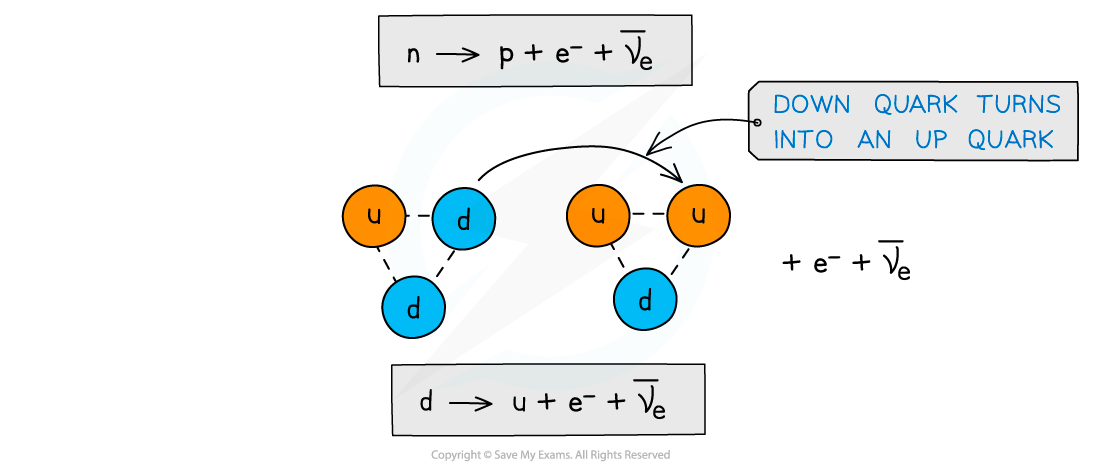Beta minus decay is when a down quark turns into an up quark

• The W boson ‘carries away’ the negative charge of the down quark which provides the negative charge for the electron and anti-neutrino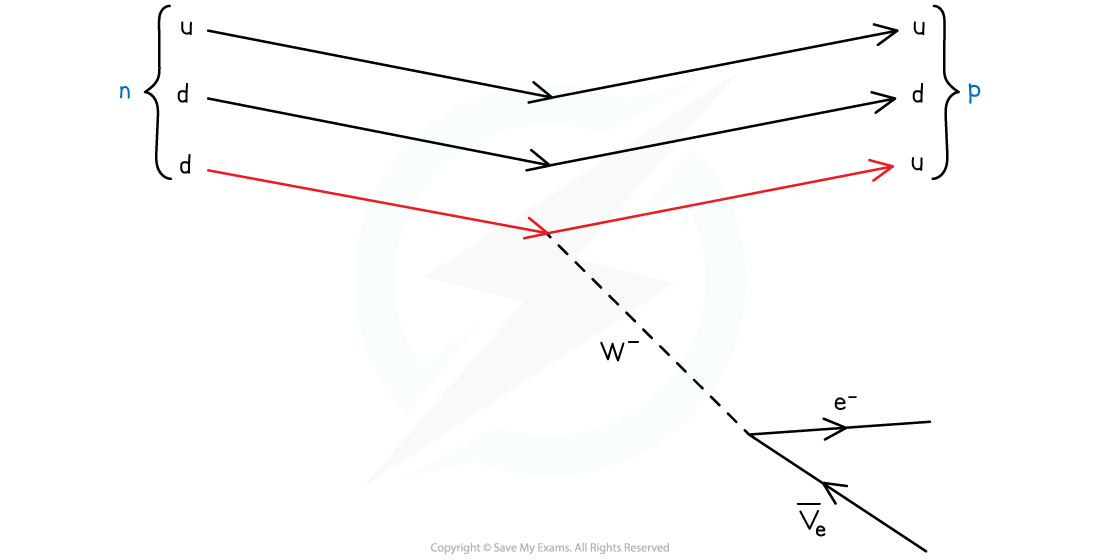In beta minus decay, the weak interaction turns a down quark into an up quark

#### Quark Composition: β+ decay

• β+ decay is when a proton turns into a neutron emitting an positron and an electron neutrino
• More specifically, a proton turns into a neutron because an up quark turns into a down quark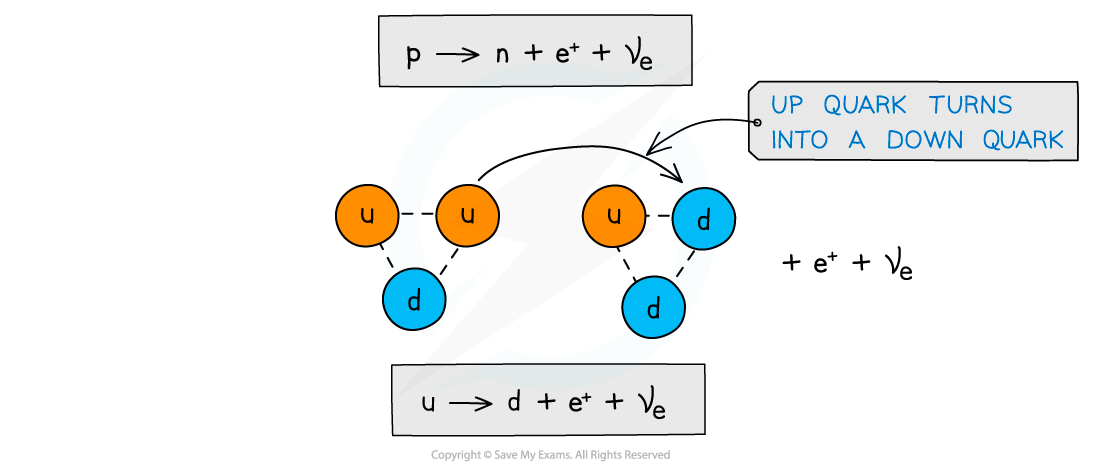Beta plus decay is when an up quark turns into a down quark

• The W+ boson ‘carries away’ the positive charge of the up quark which provides the positive charge for the positron and neutrino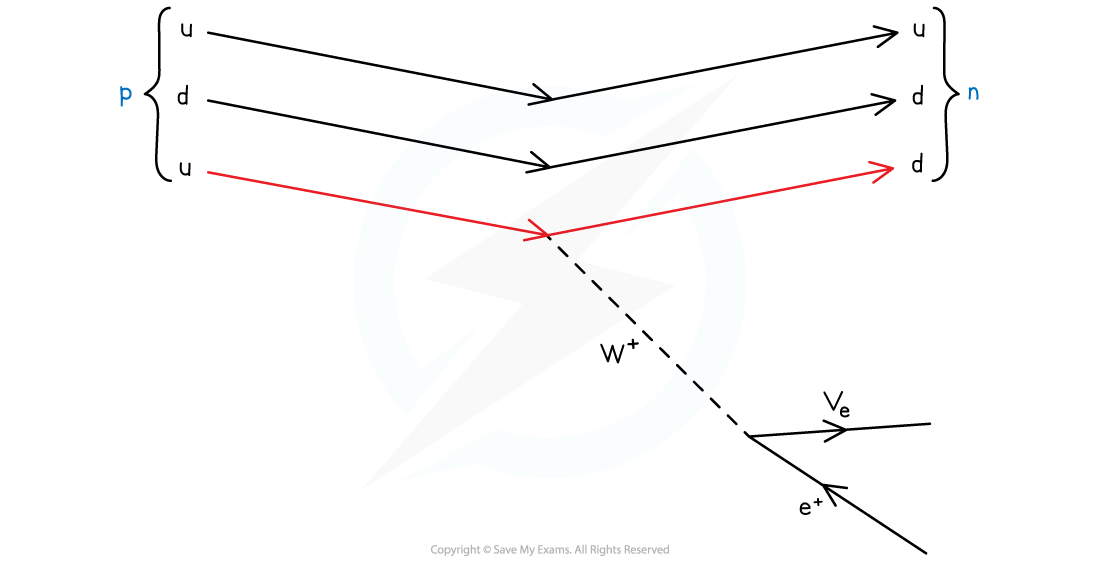In beta plus decay, the weak interaction turns an up quark into a down quark

### Confinement

• Quarks are only allowed to exist within hadrons: baryons and mesons
• Quarks cannot be alone and isolated
• Quarks exchange gluons to keep them in place relative to each other
• Attempting to separate quarks from their hadrons produces yet more hadrons using the energy required to separate them
• This is known as quark confinement
• When quarks are moved apart the energy required to do so increases rapidly
• For example, when pulling the quark-antiquark pair of a meson apart
• As they are pulled apart the energy builds and when the energy is high enough, it generates another quark-antiquark pair
• This quark-antiquark pair bridges the gap between the original quark-antiquark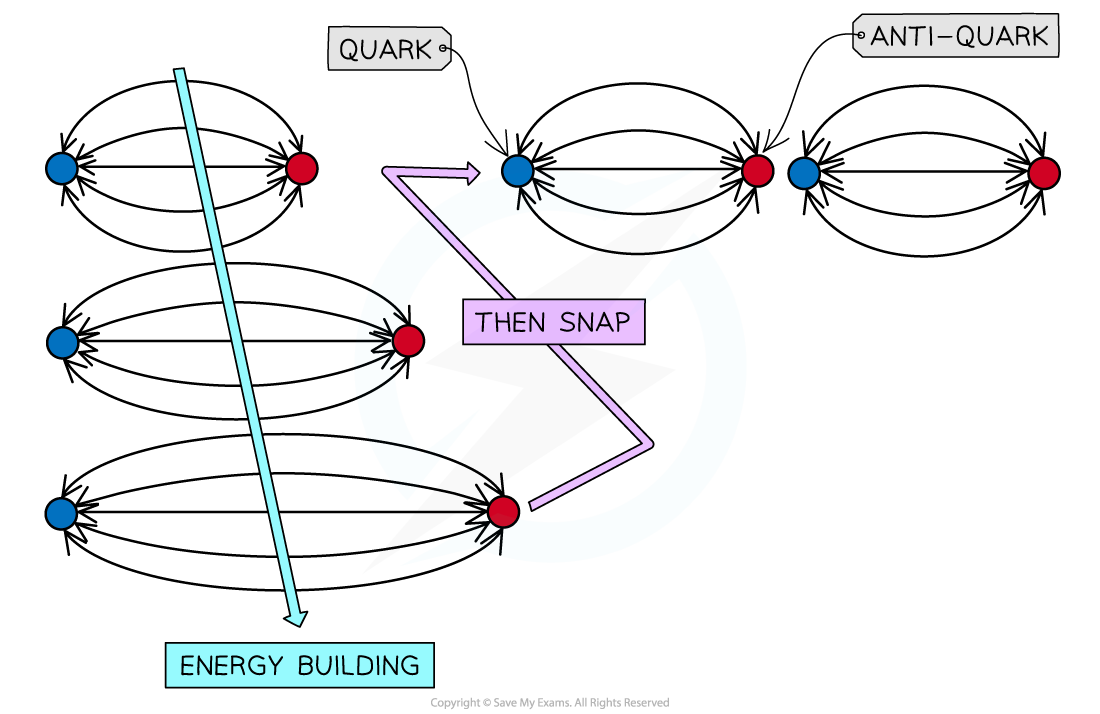A meson pulled apart will make another new meson rather than isolated quarks

• Quark confinement is part of the theory of quantum chromodynamics (QCD), the study of which is beyond the scope of the IB DP Physics syllabus